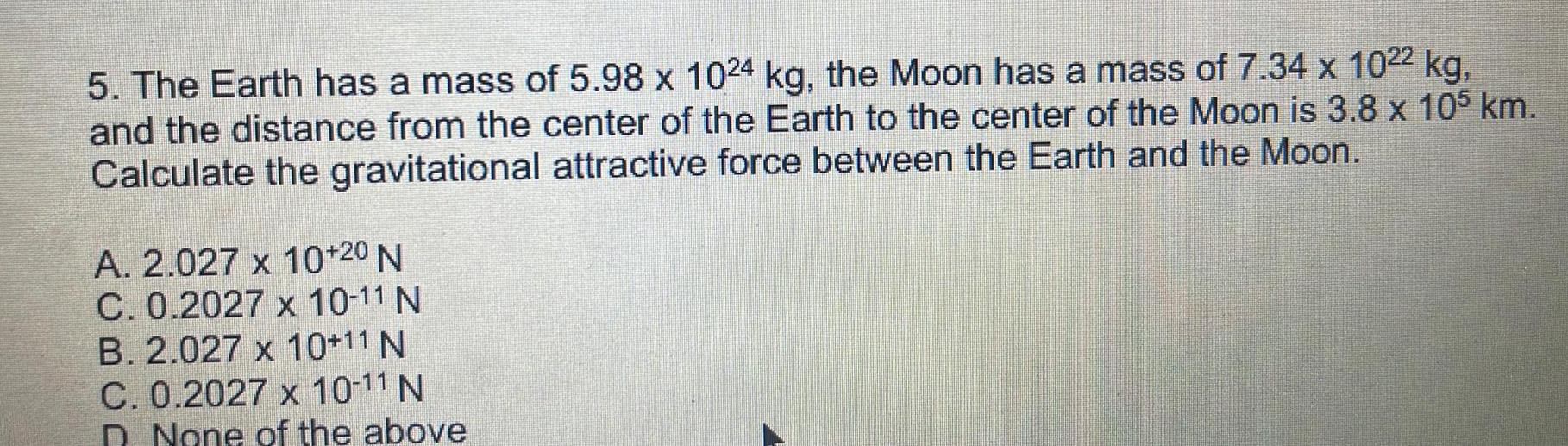Question:

# The Earth has a mass of 5.98 x 1024 kg, the Moon has a mass

Last updated: 8/9/2022The Earth has a mass of 5.98 x 1024 kg, the Moon has a mass of 7.34 x 1022 kg, and the distance from the center of the Earth to the center of the Moon is 3.8 x 105 km. Calculate the gravitational attractive force between the Earth and the Moon. A. 2.027 x 10^+20 N C. 0.2027 x 10^-11 N B. 2.027 x 10^+11 N C. 0.2027 x 10^-11 N D. None of the above